## Contravariant Tensor

A contravariant tensor is a Tensor having specific transformation properties (c.f., a Covariant Tensor). To examine the transformation properties of a contravariant tensor, first consider a Tensor of Rank 1 (a Vector)(1)

for which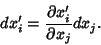(2)

Now let, then any set of quantitieswhich transform according to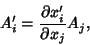(3)

or, defining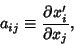(4)

according to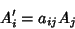(5)

is a contravariant tensor. Contravariant tensors are indicated with raised indices, i.e.,.

Covariant Tensors are a type of Tensor with differing transformation properties, denoted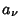. However, in 3-D Cartesian Coordinates,(6)

for, 2, 3, meaning that contravariant and covariant tensors are equivalent. The two types of tensors do differ in higher dimensions, however. Contravariant Four-Vectors satisfy(7)

whereis a Lorentz Tensor.

To turn a Covariant Tensor into a contravariant tensor, use the Metric Tensorto write(8)

Covariant and contravariant indices can be used simultaneously in a Mixed Tensor.

See also Covariant Tensor, Four-Vector, Lorentz Tensor, Metric Tensor, Mixed Tensor, Tensor

References

Arfken, G. Noncartesian Tensors, Covariant Differentiation.'' §3.8 in Mathematical Methods for Physicists, 3rd ed. Orlando, FL: Academic Press, pp. 158-164, 1985.

Morse, P. M. and Feshbach, H. Methods of Theoretical Physics, Part I. New York: McGraw-Hill, pp. 44-46, 1953.